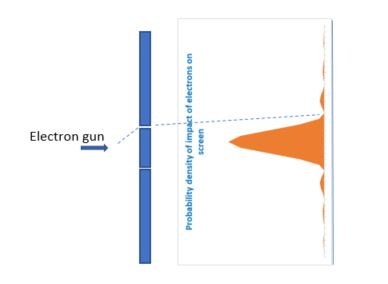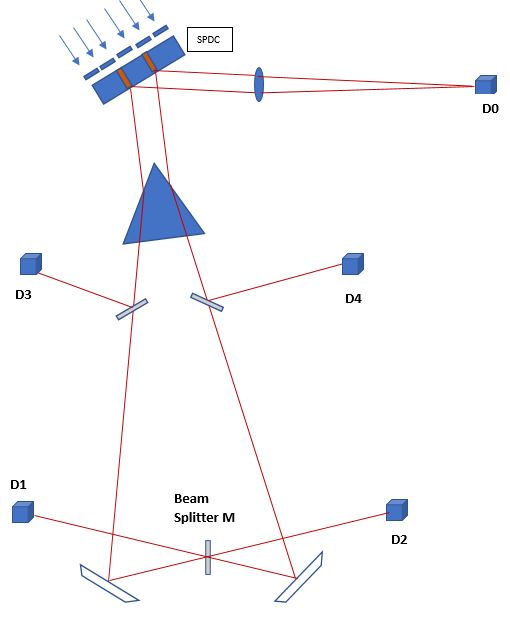# Can a quantum particle come to a fork in the road and take it?

I have always been fascinated by the weirdness of the Universe. One aspect of the weirdness is the quantum nature of things – others relate to the mysteries of Lorentz invariance, Special Relativity, the General Theory of Relativity, the extreme size and age of the Universe, the vast amount of stuff we don't seem to be able to see and so on.

This post is about an experiment that directly points to the fundamental weirdness of small (and these days, not so small) particles. While quantum effects matter at the sub-atomic particle level, these effects can coalesce into macroscopic phenomena like superconductivity, the quantum Hall effect and so on, so they can't be ignored. This experiment, usually referred to as the "Double-Slit" experiment, is described and explained in detail in Vol. 3 of the Feynman Lectures in Physics. While it would be silly of me to try and outdo Feynman's explanation, which by the way was one of the reasons why I was enthused to study physics in the first place,  I want to go beyond the Double-Slit experiment to discuss the Delayed-Choice experiment – this extra wrinkle on the Double-Slit experiment was invented by the famous scientist John Wheeler (who was Feynman's Ph.D advisor) and displays for all to see, even more aspects of quantum weirdness.

Let's get started.

The Double-Slit experiment  is carried out by shooting electrons at a pair of closely placed slits – the electron flux is sufficiently small that one is able to count the number of times electrons hit various points on a television screen placed past the slits. If no measures are taken to identify which of the two paths the electrons actually took to reach the screen, then the probability density of arrival at various points on the television screen displays an “interference'' pattern. If, however, the experiment is set up so as to identify which slit the electron went through, for example by shining an intense beam of photons at the slits that scatter off the electrons, then the interference pattern for those “which-path'' identified electrons switches to a “clump'' pattern, centered around the open slit. The standard experiment is displayed, schematically, below, where since both slits are open and we don't bother to check which slit the electron goes through, we see an "interference" pattern. If we used photons (light) instead of electrons, we'd see alternate light and dark fringes.If only one slit were open, we'd get a "clump" pattern as belowNote – no interference "bumps" at places far away from the peak.

This behavior is also what we'd get for light – photons.

Quantum mechanics is the theory that was constructed to "explain" this behavior. We construct a quantity called the "amplitude". The "amplitude" is a complex number that has a (complex) value at every point in space and time. Complex numbers have two properties – a magnitude and a phase. The magnitude squared of the amplitude at some time$t$  times a small volume in space$v$  is the probability of finding the particle (if its an amplitude for the electron, then the probability of finding the electron etc.) in that volume$v$ at time$t$. Since you need to multiply the magnitude squared by a little volume element, the squared magnitude of the amplitude is referred to as the "Probability Density".

Schrodinger's equation writes down how this amplitude evolves in time – from the electron gun to the screen. To this equation, you need to add the "Born" prescription – that you have to square the magnitude of the amplitude to get the probability density.

Feynman found a neat, equivalent interpretation of Schrodinger's equation – his method basically said – if you want to find the amplitude for the electron (say) at some point in the screen, just write down path-amplitudes for all the different ways the electron could get from the electron gun to the screen. Add these path-amplitudes and then call the net sum the "total" amplitude for the electron to be found at the particular spot on the screen. Square the magnitude of this "total" amplitude and you will get the probability density for the electron to be found at that spot (times the little volume around that spot will give you the probability to find the electron at that spot).

All this discussion of path-amplitudes would be academic if the amplitudes were real numbers. The phase is a critical piece of the amplitude. Though the magnitude (squared) is the physical quantity (related to a probability), the magnitude of a sum of complex numbers depends delicately on the phase difference between the two. As an example,$z_1=1+2i, z_2=-1-2i, z_3=1-2i, z_4=-1+2i$ have the same magnitude$\sqrt{5}$. However,$z_1+z_2=0, z_1+z_3=2, z_1+z_4=4i$ all of which have magnitudes very different from just the sum of the individual magnitudes. That's the reason we get alternate light and dark fringes on the television screen – the phases of the amplitudes for the electron to get to those spots from either of the two slits sometimes causes the sum amplitude to be 0 (which is called destructive interference) and sometimes causes the sum amplitude to add to the maximum (which is called constructive interference) and all magnitudes between these two extremes. While this behavior is extremely counter-intuitive for particles, it resembles behavior we are used to with waves, as this YouTube video shows, so does this one. This is usually referred to as wave-particle duality.

The thing you need to take away from this experiment is that if you don't force the electron to get to the screen through only one slit, by say, closing off the other slit, it appears to behave like it goes through both slits.

Wait, it gets even more interesting.

The Delayed-Choice experiment was proposed by Marlan Scully (who is an inspiring scientist in his own right) and is portrayed in the accompanying figure.An SPDC (spontaneous parametric down conversion) setup is used – basically, each of the red regions in the picture above produces two photons, when one photon hits it. Since the laser's photon can go through either of the two slits, this is just like the Double-Slit experiment, except that things are arranged so that after going through a slit, the photon would produce two other photons. The photons that travel towards the interferometer/detector$D0$ are referred to as “signal'' photons. The photons that travel towards the prism are the “idler'' photons. After passing through the prism, the “idler'' photons pass through a beam-splitter, that has a$50 \%$ probability of deflecting the incoming photon to detectors$D3$ and$D4$ respectively and$50\%$ probability of letting the photon pass on to the fully silvered (reflecting mirrors) at the bottom of the picture. Another beam-splitter is placed between the detectors$D1$ and$D2$, so photons that are detected at$D1$ and$D2$ have their “which-path'' information obliterated – for instance, an “idler'' photon arriving at$D1$ could have come along either of two paths. The actual experiment was performed by Kim et. al.

The detector$D0$ accumulates “signal'' photons – a coincidence counter correlates it to “idler'' photons detected at the detectors$D3, D4, D1$ and$D2$ (the “idler'' photons arrive at those detectors a few nanoseconds after the “signal'' photons are received. From the accumulated “signal'' photons received at$D0$, if we separate the ones received in coincidence with the detectors$D3$ or$D4$, since the “which-path'' information is clear in those cases, the pattern of interference observed (in the spatial density of the “signal'' photons) is the “clump'' pattern. However, the accumulated “signal'' photons received at$D0$ that are coincident with the ones received at$D1$ display an interference pattern, since one cannot infer the path taken by the “idler'' photons that show up at detector$D1$, which means one cannot tell which slit the “signal'' photon came through before arriving at detector$D0$. Similarly, the accumulated “signal'' photons received at$D0$ that are coincident with the ones received at$D2$ display an interference pattern in their spatial distribution, however this pattern is spatially offset half a wavelength off the one due to$D1$. This is just enough to put peaks where the other pattern has dips and vice versa. So, if you aren't careful to note which detector$D1$ or$D2$ detected the photon coincident with the "signal" photon detector$D0$, you get a clump pattern. The reason for this offset is  little tricky to explain – its called "unitarity" or conservation of probability at the beam-splitter, which forces some delicate phase assignments for the amplitudes we spoke about earlier.

Note, however, that someone could hide the specific arrival times of the photons at$D1$ and$D2$ from you for months and then tell you, say, a year later. All this time, you wouldn't have known there was an interference pattern "hiding" under the massive "clump" pattern you see. When you selectively look at the coincident detections separately for$D1$ and$D2$, it is then and only then, that you see the interference pattern.

Curious! This experiment, as I said, has been done and the results are as described above.

With a friend, I put together another set up in an interesting locale, a black hole,  that we tried to make work. Quantum mechanics defeated us in our attempts too, but its an interesting problem to work through.

1.Skanda Rao says:
1.Simply Curious says:
The SPDC crystal is non-linear and has a molecule with energy levels such that occasionally two photons are emitted from the same point in the crystal when hit by a photon of double the energy. Since this needs a double interaction to take place, it happens much less often – often for every$10^{12}$ photons, you will produce one pair of half-energy/double wavelength child photons. Of course, this is a large number. but remember a 100 watt bulb emits around$10^{20}$ photons per second, so this isn’t a big deal for a powerful laser.